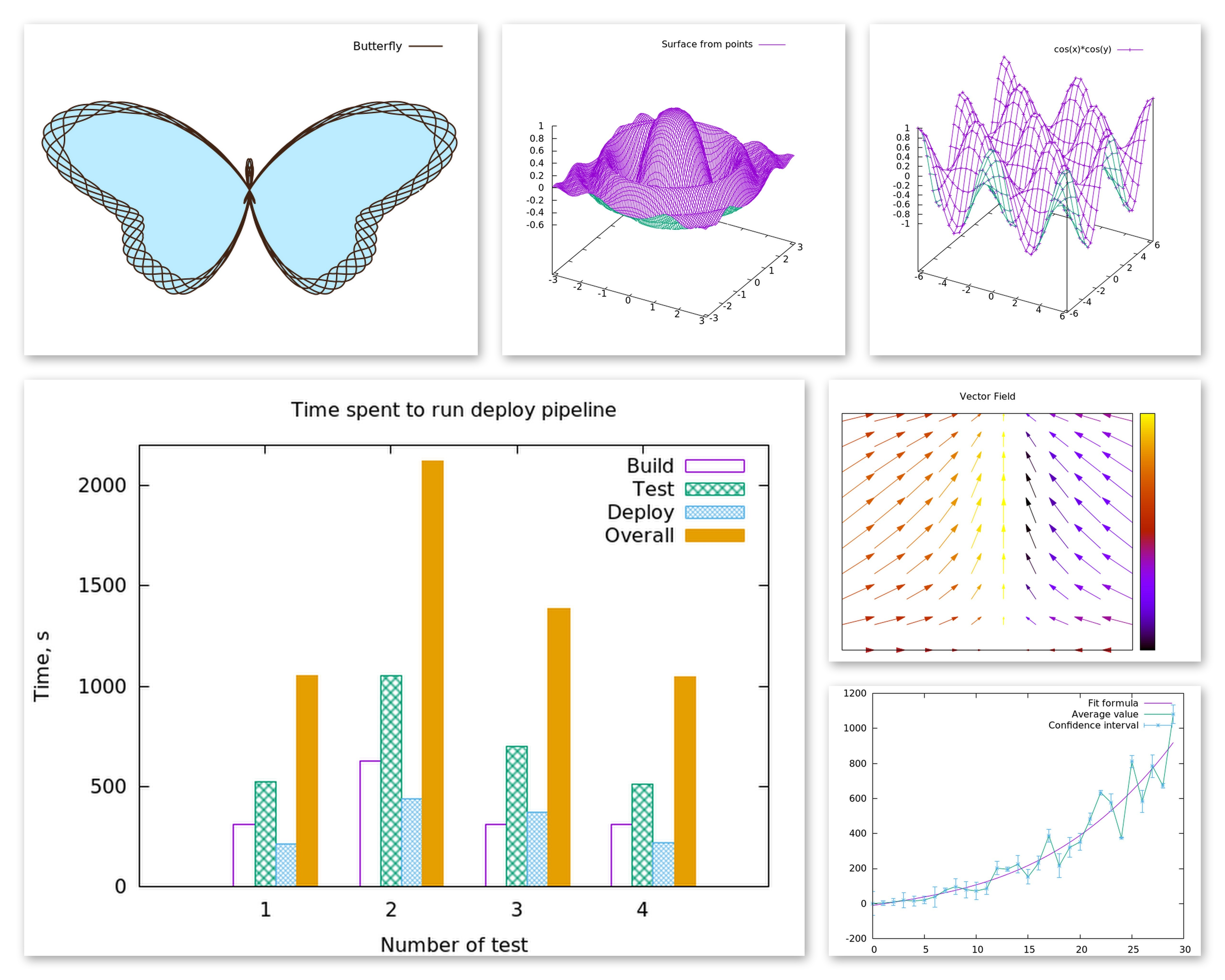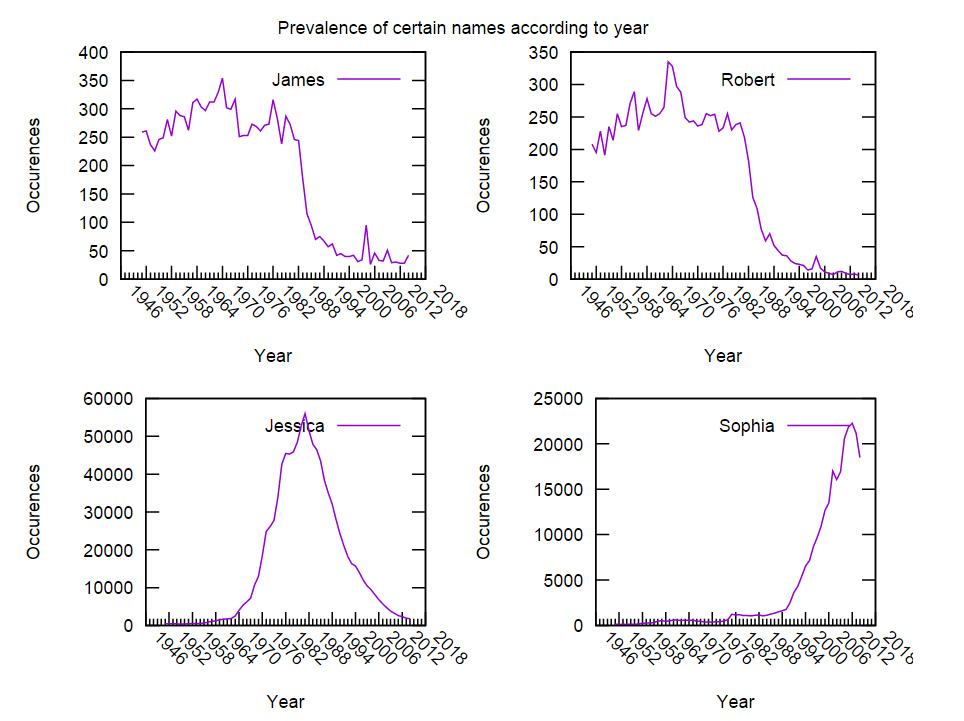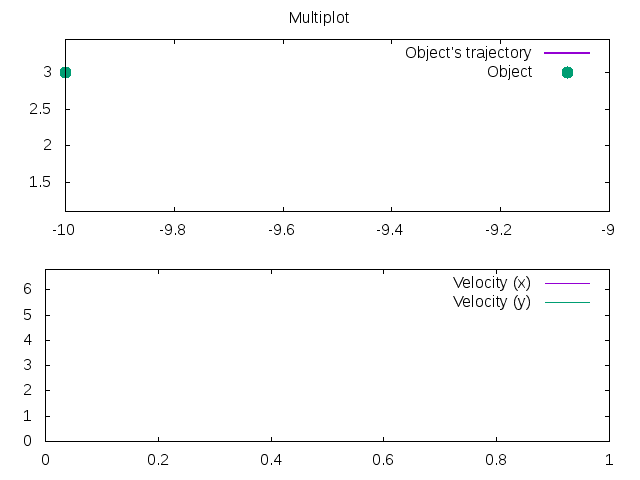# GnuplotRB and GSoC 2015

## Introduction

This summer I’ve been participating in Google Summer of Code with GnuplotRB project (plotting tool for Ruby users based on Gnuplot) for SciRuby. GSoC is almost over and I’m releasing v0.3.1 of GnuplotRB as a gem. In this blog post I want to introduce the gem and highlight some of its capabilities.

## Features

There are several existing plotting tools for Ruby such as Nyaplot, Plotrb, Rubyvis and Gnuplot gem. However they are not designed for large datasets and have fewer plotting styles and options than Gnuplot. Gnuplot gem was developed long ago and nowadays consists mostly of hacks and does not support modern Gnuplot features such as multiplot.

Therefore my goal was to develop new gem for Gnuplot which would allow full use of its features in Ruby. I was inspired to build an easy-to-use interface for the most commonly used features of Gnuplot and allow users to customize their plots with Gnuplot options as easily as possible in Rubyesque way.

### 2D and 3D plots

The main feature of every plotting tool is its ability to plot graphs. GnuplotRB allows you to plot both mathematical formula and (huge) sets of data. GnuplotRB supports plotting 2D graphs (`GnuplotRB::Plot` class) in Cartesian/parametric/polar coordinates and 3D graphs (`GnuplotRB::Splot` class) — in Cartesian/cylindrical/spherical coordinates.

There are vast of plotting styles supported by GnuplotRB:

• `points`
• `lines`
• `histograms`
• `boxerrorbars`
• `circles`
• `boxes`
• `filledcurves`
• `vectors`
• `heatmap`
• etc (full list in gnuplot doc p. 47)

Plot examples:For code examples please see the repository README, notebooks and the examples folder.

### Multiplot

`GnuplotRB::Multiplot` allows users to place several plots on a single layout and output them at once (e.g., to a PNG file). Multiplot notebook.

Here is a multiplot example (taken from Sameer’s notebook):### Animated plots

GnuplotRB may output any plot to gif file but `GnuplotRB::Animation` allows to make this gif animated. It takes several `Plot` or `Splot` objects just as multiplot does and outputs them one-by-one as frames of gif animation. Animation notebook.### Fit

Although the main GnuplotRB’s purpose is to provide you with swift, robust and easy-to-use plotting tool, it also offers a `Fit` module that contains several methods for fitting given data with a function. See examples in Fit notebook.

### Integration with other SciRuby tools

#### Embedding plots into iRuby notebooks

GnuplotRB plots may be embedded into iRuby notebooks as JPEG/PNG/SVG images, as ASCII art or GIF animations (`Animation` class). This functionality explained in a special iRuby notebook.

#### Using data from Daru containers

To link GnuplotRB with other SciRuby tools I implemented plot creation from data given in Daru containers (`Daru::Dataframe` and `Daru::Vector`). One can use `daru` gem in order to work with statistical SciRuby gems and plotting with GnuplotRB. Notebooks with examples: 1, 2.

### Possible datasources for plots

You can pass to Plot (or Splot or Dataset) constructor data in the following forms:

• String containing mathematical formula (e.g., `'sin(x)'`)
• String containing name of file with data (e.g., `'points.data'`)
• Some Ruby object responding to `#to_gnuplot_points`
• `Array`
• `Daru::Dataframe`
• `Daru::Vector`

See examples in notebooks.